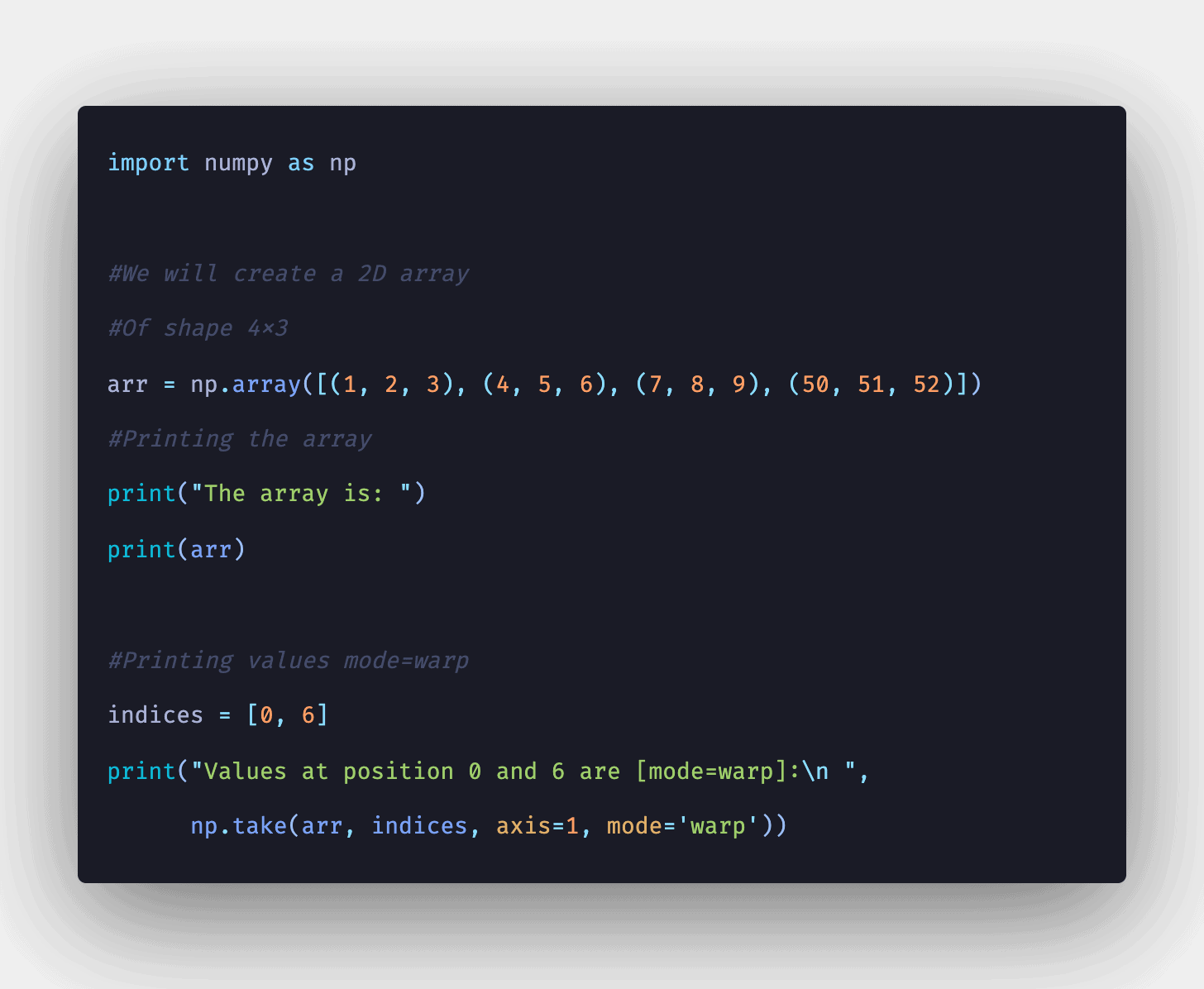# Python NumPy take() Function ExamplePython NumPy take() is an inbuilt NumPy function that is used to return elements from an array along the mentioned axis and indices.

Python NumPy take() is an inbuilt NumPy function that is used to return elements from an array along the mentioned axis and indices. Means, we will be able to get elements of an array by its indices, and if the axis mentioned, then all elements present at that index will be printed axis wise.

### Python NumPy take()

Python NumPy take() function takes elements from an array along an axis. The take() function does a same thing as “fancy” indexing (indexing arrays using arrays); however, it can be easier to use if you need items along the given axis.

#### Syntax

``numpy.take(array, indices, axis = None, out = None, mode =’raise’)``

## Python Tricks Every Developer Should Know

In this tutorial, you’re going to learn a variety of Python tricks that you can use to write your Python code in a more readable and efficient way like a pro.

## How to Remove all Duplicate Files on your Drive via Python

Today you're going to learn how to use Python programming in a way that can ultimately save a lot of space on your drive by removing all the duplicates. We gonna use Python OS remove( ) method to remove the duplicates on our drive. Well, that's simple you just call remove ( ) with a parameter of the name of the file you wanna remove done.

## NumPy Array Tutorial - Python NumPy Array Operations and Methods

Learn about NumPy Array, NumPy Array creation, various array functions, array indexing & Slicing, array operations, methods and dimensions,It also includes array splitting, reshaping, and joining of arrays. Even the other external libraries in Python relate to NumPy arrays.

## NumPy in Python | NumPy Python Tutorial | Python Programming

NumPy in Python explains what exactly is Numpy and how it is better than Lists. It also explains various Numpy operations with examples.

## Basic Data Types in Python | Python Web Development For Beginners

In the programming world, Data types play an important role. Each Variable is stored in different data types and responsible for various functions. Python had two different objects, and They are mutable and immutable objects.